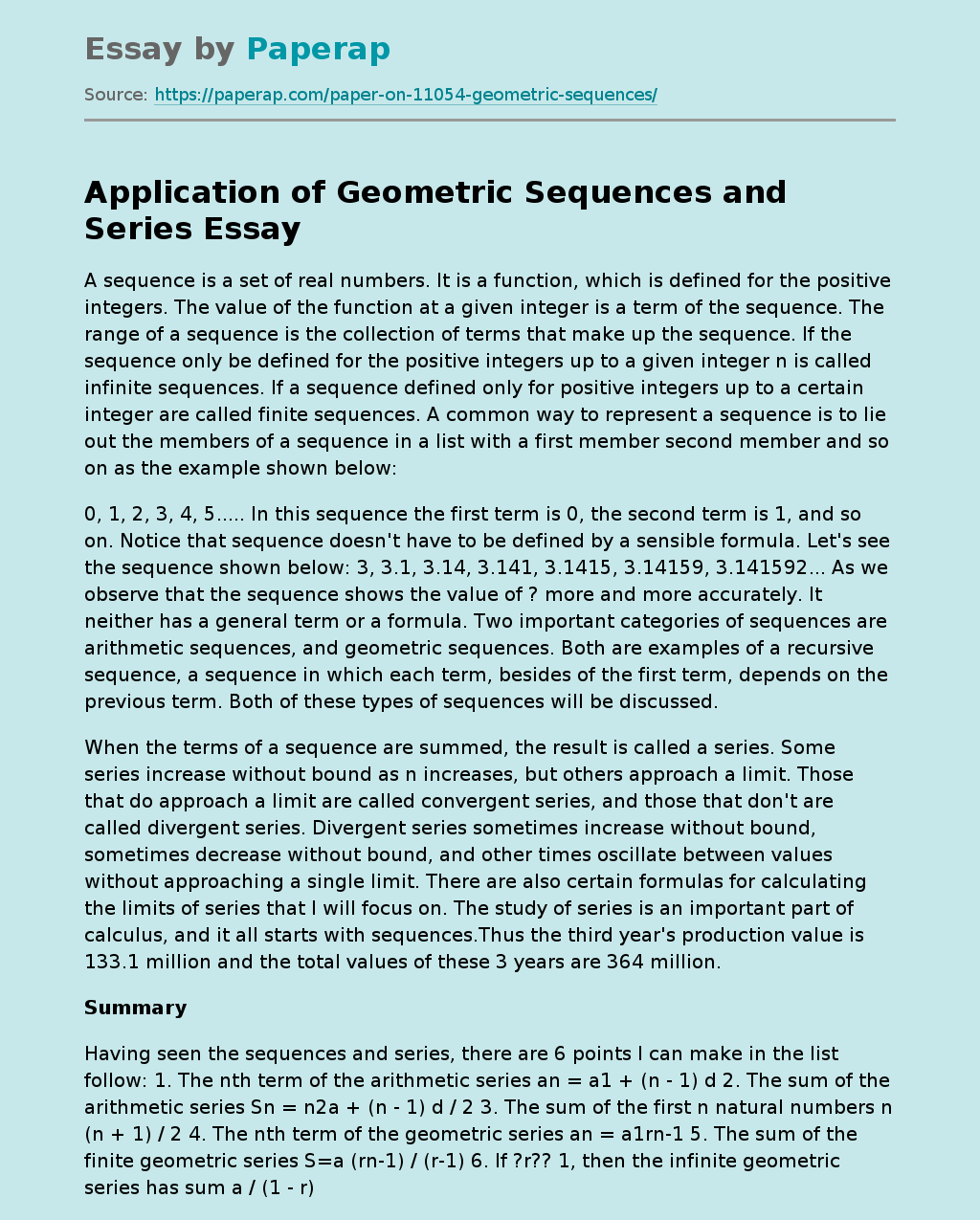# Application of Geometric Sequences and Series

A sequence is a set of real numbers. It is a function, which is defined for the positive integers. The value of the function at a given integer is a term of the sequence. The range of a sequence is the collection of terms that make up the sequence. If the sequence only be defined for the positive integers up to a given integer n is called infinite sequences. If a sequence defined only for positive integers up to a certain integer are called finite sequences.

A common way to represent a sequence is to lie out the members of a sequence in a list with a first member second member and so on as the example shown below:

0, 1, 2, 3, 4, 5….. In this sequence the first term is 0, the second term is 1, and so on. Notice that sequence doesn’t have to be defined by a sensible formula. Let’s see the sequence shown below: 3, 3.1, 3.14, 3.141, 3.1415, 3.14159, 3.141592… As we observe that the sequence shows the value of ? more and more accurately.

It neither has a general term or a formula. Two important categories of sequences are arithmetic sequences, and geometric sequences. Both are examples of a recursive sequence, a sequence in which each term, besides of the first term, depends on the previous term. Both of these types of sequences will be discussed.

When the terms of a sequence are summed, the result is called a series. Some series increase without bound as n increases, but others approach a limit. Those that do approach a limit are called convergent series, and those that don’t are called divergent series.

Get quality help nowSweet VVerified

Proficient in: Calculus4.9 (984)

“ Ok, let me say I’m extremely satisfy with the result while it was a last minute thing. I really enjoy the effort put in. ”+84 relevant experts are online

Divergent series sometimes increase without bound, sometimes decrease without bound, and other times oscillate between values without approaching a single limit. There are also certain formulas for calculating the limits of series that I will focus on. The study of series is an important part of calculus, and it all starts with sequences.Thus the third year’s production value is 133.1 million and the total values of these 3 years are 364 million.

## Summary

Having seen the sequences and series, there are 6 points I can make in the list follow: 1. The nth term of the arithmetic series an = a1 + (n – 1) d 2. The sum of the arithmetic series Sn = n2a + (n – 1) d / 2 3. The sum of the first n natural numbers n (n + 1) / 2 4. The nth term of the geometric series an = a1rn-1 5. The sum of the finite geometric series S=a (rn-1) / (r-1) 6. If ?r?? 1, then the infinite geometric series has sum a / (1 – r)